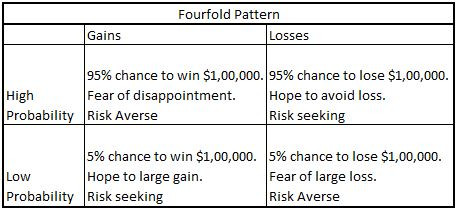Search
•Kalpesh Agrawal

# CM2 Topics

List of CM2 topics.

The dominant theory

Fundamental analysis

Efficient Market Hypothesis (EMH)

• Weak form EMH

• Semi-strong form EMH

• Strong form EMH

Informational efficiency

Volatility tests

Meaning of utility

Utility functions

The expected utility theorem

The marginal utility of wealth

Fair gamble

Derivation of the expected utility theorem

• Comparability

• Transitivity

• Independence

• Certainty equivalence

Non-satiation (Utility function = U'(w) > 0)

Risk aversion

• Risk-averse investor (Utility function = U''(w) < 0)

• Risk-seeking investor (Utility function = U''(w) > 0)

• Risk-neutral investor (Utility function = U''(w) = 0)

Measuring risk aversion

Take one example of a fair gamble represented by a random variable y. The amounts that an investor can lose or win are fixed absolute amounts. In this case, the amount lost or won by the investor is independent of the wealth (w).

So, if the investor accepts the gamble, the resulting final wealth is w + y.

U(Cw) = E[U(w + x)]

Multiplicative/proportional gamble:

Take one example of a fair gamble represented by a random variable y. The amounts that an investor can lose or win are all expressed as proportions of the initial wealth.

So, if the investor accepts the gamble, the resulting wealth is w * y.

U(Cw) = E[U(w * y)]

Absolute risk aversion (ARA)

• denoted by A(w)

• measured by the function A(w) = -U''(w) / U'(w)

Relative risk aversion

• denoted by R(w)

• measured by the function R(w) = -w * (-U''(w) / U'(w))

The log utility function

Iso-elastic utility function

The power utility function

Negative exponential utility function

Hyperbolic Absolute Risk Aversion (HARA)

State-dependent utility functions

How to find maximum and minimum premium

Limitations of utility theory

What is Behavioural finance?

Absolute dominance

Stochastic dominance

• First-order stochastic dominance

• Second-order stochastic dominance

Prospect theory:

Daniel Kahneman and Amos Tversky developed a 'Prospect Theory' in 1979.

In this paper, they stated that people would like to evaluate gains and losses differently.

Kahneman conducted more research in loss aversion and discovered several additional key insights.

The insights captured by him are called the Fourfold Pattern, which goes beyond loss aversion to show the di¤erent ways people respond to high-probability and low-probability events when they are either gains or losses.

This is the answer to "Why Insurance exists"!!!

Even though the chance of a loss is small, if it happens it will be painful. Hence we're willing to pay a small insurance premium to eliminate or reduce the risk.